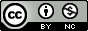Produced in R version 3.6.1.

• Suppose we want to know the statistical behavior of the estimator $$\hat\theta({y_{1:N}})$$ for models in a neighborhood of the MLE, $$\theta^*=\hat\theta(y_{1:N}^*)$$.

• In particular, let’s consider the problem of estimating uncertainty about $$\theta_1$$. We want to assess the behavior of the maximum likelihood estimator, $$\hat\theta({y_{1:N}})$$, and possibly the coverage of an associated confidence interval estimator, $$\big[\hat\theta_{1,\mathrm lo}({y_{1:N}}),\hat\theta_{1,\mathrm hi}({y_{1:N}})\big]$$. The confidence interval estimator could be constructed using either the Fisher information method or the profile likelihood approach.

• The following simulation study lets us address the following goals:

1. Evaluate the coverage of a proposed confidence interval estimator, $$[\hat\theta_{1,\mathrm lo},\hat\theta_{1,\mathrm hi}]$$,

2. Construct a standard error for $$\theta_1^*$$,

3. Construct a confidence interval for $$\theta_1$$ with exact local coverage.
1. Generate $$J$$ independent Monte Carlo simulations, $Y_{1:N}^{[j]} \sim f_{Y_{1:N}}(y_{1:N}\, ; \,\theta^*)\mbox{ for } j\in 1:J.$

2. For each simulation, evaluate the maximum likelihood estimator, $\theta^{[j]} = \hat\theta\big(Y_{1:N}^{[j]}\big)\mbox{ for } j\in 1:J,$ and, if desired, the confidence interval estimator, $\big[\theta^{[j]}_{1,\mathrm lo},\theta^{[j]}_{1,\mathrm hi}\big] = \big[\hat\theta_{1,\mathrm lo}({X^{[j]}_{1:N}}),\hat\theta_{1,\mathrm hi}({X^{[j]}_{1:N}})\big].$

3. We can use these simulations to obtain solutions to our goals for uncertainty assessment:

1. For large $$J$$, the coverage of the proposed confidence interval estimator is well approximated, for models in a neighborhood of $$\theta^*$$, by the proportion of the intervals $$\big[\theta^{[j]}_{1,\mathrm lo},\theta^{[j]}_{1,\mathrm hi}\big]$$ that include $$\theta_1^*$$.

2. The sample standard deviation of $$\{ \theta^{[j]}_1, j\in 1:J\}$$ is a natural standard error to associate with \$.

3. For large $$J$$, one can empirically calibrate a 95% confidence interval for $$\theta_1$$ with exactly the claimed coverage in a neighborhood of $$\theta^*$$. For example, using profile methods, one could replace the cutoff 1.92 by a constant $$\alpha$$ chosen such that 95% of the profile confidence intervals computed for the simulations cover $$\theta_1^*$$.

Back